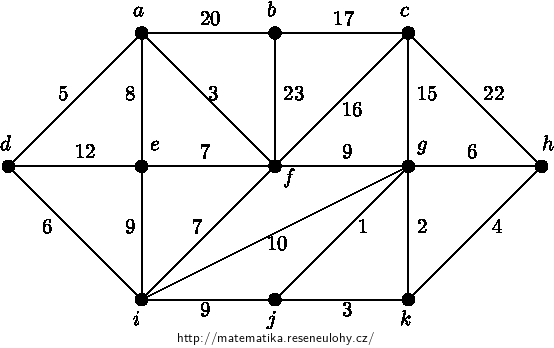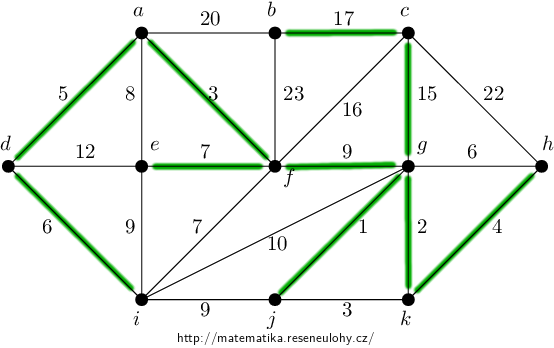## Minimal spanning tree

Determine the minimum spanning tree for the depicted graph:• #### Solution

We sort the edges according to the weights in ascending order. We arrange the edges of the same weights arbitrarily, but this can lead to a different solution. One of the possible sequences starts with the edges $$(g, j)$$ - 1, $$(g, k)$$ - 2, $$(j, k)$$ - 3 , $$(a, f)$$ - 3,…

According to this sequence, we select the edges into the spanning tree, provided that it does not create a cycle with the previously selected edges. Thus, the first edge that will not be selected into the spanning tree is the edge $$(j, k)$$, because with the previously selected edges $$(g, j)$$ and $$(g, k )$$ created a cycle on the vertices $$g, j, k$$.

Follow this procedure until the selected edges form a connected graph – a spanning tree.The alternative solution has the edge $$(i, j)$$ instead of the edge $$(f, g)$$.